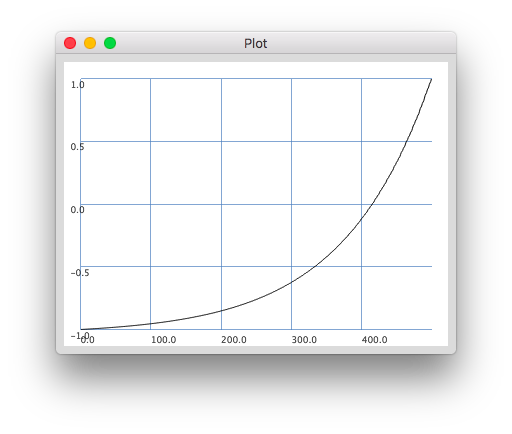# How to plot a transfer function?

Hello

Again, a simple question.
I was wondering if I could use lincurve for negative numbers, and thus if I could plot the transfer function, with values from -1.0 to 1.0 on the x-axis, and `x.lincurve(-1.0, 1.0, -1.0, 1.0, 4)` on the y-axis.

``````{ |x| x.lincurve(-1,1,-1,1,4) }.plotGraph(from: -1, to: 1));
``````does more or less what I want, plot the transfer function, but the x-axis displays the number of values (500, the default for plotGraph), not the x-axis values I wanted.

Any idea to set to the x-axis values in the plot window ?

Thanks !

Geoffroy

Thanks James ! You pointed me in the right direction !

The problem is that plotGraph does not return a Plotter, but the function it was called on.

For future reference, how to make it work :

``````(
var func, n=500, from, to, res, array, plot;
from = -1.0;
to = 1.0;
func = { |x| x.lincurve(-1,1,-1,1,4) };
array = Array.interpolation(n, from, to);
res = array.collect { |x| func.value(x) };
plot = res.plot.domainSpecs_([from, to, \lin].asSpec).refresh
)
``````

Have a good day !

That might be considered a bug. For everything else in `Common\GUI\PlusGUI\Math\PlotView.sc` the `plot` returns the `Plotter`. I don’t quite buy that it needs to be special for `plotGraph` and return the function, but maybe it was intended to work like `trace` so you can chain-plot the function and still use it in the same expression. But unlike for `trace` that doesn’t seem incredibly useful for `plotGraph`… especially since/if you need to customize the axis, as you found.

By the way, the fact that `Function.plot` creates a synth and plots that can also be confusing. Some “smarts” to detect if it creates a UGen or not could be implemented, and plot locally (i.e. plotGraph) when there’s no UGen [instead of bombing with an obscure error, while still popping a window].

I agree. It looks like someone just forgot the `^`. Totally fine to file a bug report for that.

hjh

1 Like